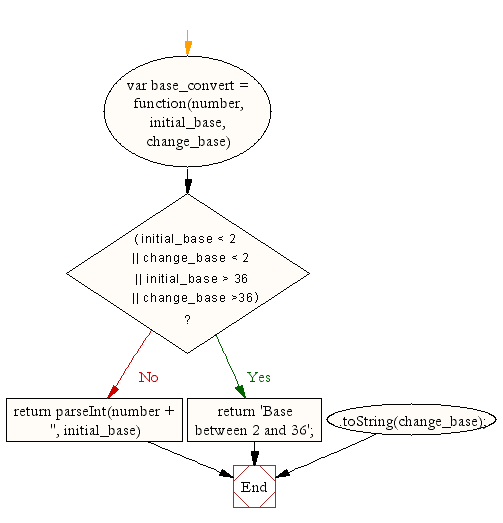# JavaScript: Convert a number from one base to another

## JavaScript Math: Exercise-1 with Solution

Write a JavaScript function to convert a number from one base to another.

Sample Solution:-

HTML Code:

``````<!DOCTYPE html>
<html>
<meta charset="utf-8">
<title>Base convert</title>
<body>

</body>
</html>
```
```

JavaScript Code:

``````var base_convert = function(number, initial_base, change_base) {
if ((initial_base && change_base) <2 || (initial_base && change_base)>36)
return 'Base between 2 and 36';

return parseInt(number + '', initial_base)
.toString(change_base);
}

console.log(base_convert('E164',16,8));
console.log(base_convert(1000,2,8));
```
```

Sample Output:

```160544
10
```

Flowchart:Live Demo:

See the Pen javascript-math-exercise-1 by w3resource (@w3resource) on CodePen.

Improve this sample solution and post your code through Disqus

What is the difficulty level of this exercise?

Test your Programming skills with w3resource's quiz.

﻿